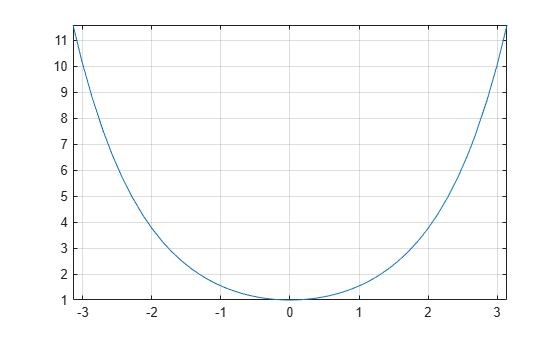# cosh

Symbolic hyperbolic cosine function

## Syntax

``cosh(X)``

## Description

example

````cosh(X)` returns the hyperbolic cosine function of `X`.```

## Examples

### Hyperbolic Cosine Function for Numeric and Symbolic Arguments

Depending on its arguments, `cosh` returns floating-point or exact symbolic results.

Compute the hyperbolic cosine function for these numbers. Because these numbers are not symbolic objects, `cosh` returns floating-point results.

`A = cosh([-2, -pi*i, pi*i/6, 5*pi*i/7, 3*pi*i/2])`
```A = 3.7622 -1.0000 0.8660 -0.6235 -0.0000```

Compute the hyperbolic cosine function for the numbers converted to symbolic objects. For many symbolic (exact) numbers, `cosh` returns unresolved symbolic calls.

`symA = cosh(sym([-2, -pi*i, pi*i/6, 5*pi*i/7, 3*pi*i/2]))`
```symA = [ cosh(2), -1, 3^(1/2)/2, -cosh((pi*2i)/7), 0]```

Use `vpa` to approximate symbolic results with floating-point numbers:

`vpa(symA)`
```ans = [ 3.7621956910836314595622134777737,... -1.0,... 0.86602540378443864676372317075294,... -0.62348980185873353052500488400424,... 0]```

### Plot Hyperbolic Cosine Function

Plot the hyperbolic cosine function on the interval from $-\pi$ to $\pi$.

```syms x fplot(cosh(x),[-pi pi]) grid on```### Handle Expressions Containing Hyperbolic Cosine Function

Many functions, such as `diff`, `int`, `taylor`, and `rewrite`, can handle expressions containing `cosh`.

Find the first and second derivatives of the hyperbolic cosine function:

```syms x diff(cosh(x), x) diff(cosh(x), x, x)```
```ans = sinh(x) ans = cosh(x)```

Find the indefinite integral of the hyperbolic cosine function:

`int(cosh(x), x)`
```ans = sinh(x)```

Find the Taylor series expansion of `cosh(x)`:

`taylor(cosh(x), x)`
```ans = x^4/24 + x^2/2 + 1```

Rewrite the hyperbolic cosine function in terms of the exponential function:

`rewrite(cosh(x), 'exp')`
```ans = exp(-x)/2 + exp(x)/2```

## Input Arguments

collapse all

Input, specified as a symbolic number, variable, expression, or function, or as a vector or matrix of symbolic numbers, variables, expressions, or functions.

## Version History

Introduced before R2006a